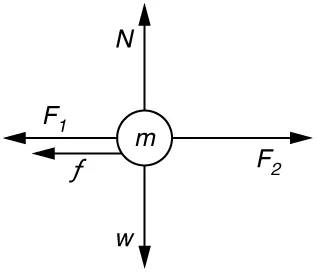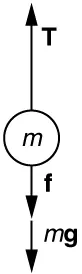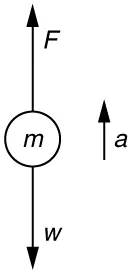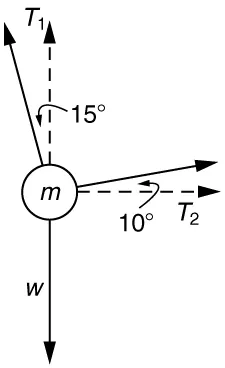College Physics 2e

# Chapter 4

### Problems & Exercises

1.

265 N

3.

$13.3 m/s 2 13.3 m/s 2$

7.

(a) $12 m/s 2 12 m/s 2$.

(b) The acceleration is not one-fourth of what it was with all rockets burning because the frictional force is still as large as it was with all rockets burning.

9.

(a) The system is the child in the wagon plus the wagon.

(b)(c) $a=0.130 m/s2a=0.130 m/s2$ in the direction of the second child’s push.

(d) $a=0.00 m/s2a=0.00 m/s2$

11.

(a) $3.68 × 10 3 N 3.68× 10 3 N$ . This force is 5.00 times greater than his weight.

(b) $3750 N; 11.3º above horizontal3750 N; 11.3º above horizontal$

13.

$1.5 × 10 3 N , 150 kg , 150 kg 1.5× 10 3 N,150 kg,150 kg$

15.

Force on shell: $2.64×107N2.64×107N$

Force exerted on ship = $−2.64×107N−2.64×107N$, by Newton’s third law

17.

(a) $0.11 m/s2 0.11 m/s2$
(b) $1.2× 10 4 N1.2× 10 4 N$

19.

(a) $7.84×10-4 N7.84×10-4 N$

(b) $1.89×10–3 N1.89×10–3 N$. This is 2.41 times the tension in the vertical strand.

21.

Newton’s second law applied in vertical direction gives

$F y = F − 2 T sin θ = 0 F y = F − 2 T sin θ = 0$
$F = 2T sin θ F = 2T sin θ$
$T = F 2 sin θ . T = F 2 sin θ .$
23.Using the free-body diagram:

$Fnet=T−f−mg=maFnet=T−f−mg=ma$,

so that

$a=T−f−mgm=1.250×107 N−4.50×106 N−(5.00×105 kg)(9.80 m/s2)5.00×105 kg=6.20 m/s2a=T−f−mgm=1.250×107 N−4.50×106 N−(5.00×105 kg)(9.80 m/s2)5.00×105 kg=6.20 m/s2$.

25.
1. Use Newton’s laws of motion.2. Given : $a=4.00g=(4.00)(9.80 m/s2)=39.2 m/s2 ; a=4.00g=(4.00)(9.80 m/s2)=39.2 m/s2 ;$$m=70.0 kgm=70.0 kg$,

Find: $FF$.

3. $∑F=+F−w=ma ,∑F=+F−w=ma ,$ so that $F=ma+w=ma+mg=m(a+g)F=ma+w=ma+mg=m(a+g)$.

$F=(70.0 kg)[(39.2 m/s2)+(9.80 m/s2)]F=(70.0 kg)[(39.2 m/s2)+(9.80 m/s2)]$$=3.43×103 N=3.43×103 N$. The force exerted by the high-jumper is actually down on the ground, but $FF$ is up from the ground and makes him jump.

4. This result is reasonable, since it is quite possible for a person to exert a force of the magnitude of $103 N103 N$.
27.

(a) $4.41×105 N4.41×105 N$

(b) $1.50×105 N1.50×105 N$

29.

(a) $910 N910 N$

(b) $1.11×103 N1.11×103 N$

31.

$a = 0.139 m/sa=0.139 m/s$, $θ = 12.4º θ=12.4º$ north of east

33.
1. Use Newton’s laws since we are looking for forces.
2. Draw a free-body diagram:3. The tension is given as $T=25.0 N.T=25.0 N.$ Find $Fapp.Fapp.$ Using Newton’s laws gives: $Σ Fy=0,Σ Fy=0,$ so that applied force is due to the y-components of the two tensions: $Fapp=2 T sin θ=2(25.0 N)sin(15º)=12.9 NFapp=2 T sin θ=2(25.0 N)sin(15º)=12.9 N$

The x-components of the tension cancel. $∑Fx=0∑Fx=0$.

4. This seems reasonable, since the applied tensions should be greater than the force applied to the tooth.
40.

$10.2 m/s 2 , 4.67º from vertical 10.2 m/s 2 , 4.67º from vertical$

42.$T 1 = 736 N T 1 = 736 N$

$T 2 = 194 N T 2 = 194 N$

44.

(a) $7.43 m/s7.43 m/s$

(b) 2.97 m

46.

(a) $4.20 m/s4.20 m/s$

(b) $29.4 m/s229.4 m/s2$

(c) $4.31×103 N4.31×103 N$

48.

(a) 47.1 m/s

(b) $2.47×103 m/s22.47×103 m/s2$

(c) $6.18×103 N6.18×103 N$ . The average force is 252 times the shell’s weight.

52.

(a) $1×10−131×10−13$

(b) $1×10−111×10−11$

54.

$10 2 10 2$

55.

(a) Box A travels faster at the finishing distance since a greater force with equal mass results in a greater acceleration. Also, a greater acceleration over the same distance results in a greater final speed.

(b) i. Yes, it is consistent because a greater force results in a greater final speed. ii. It does not make sense because $V= 2(f/m)x =K (F) V= 2(f/m)x =K (F)$.

(c)

Order a print copy

As an Amazon Associate we earn from qualifying purchases.